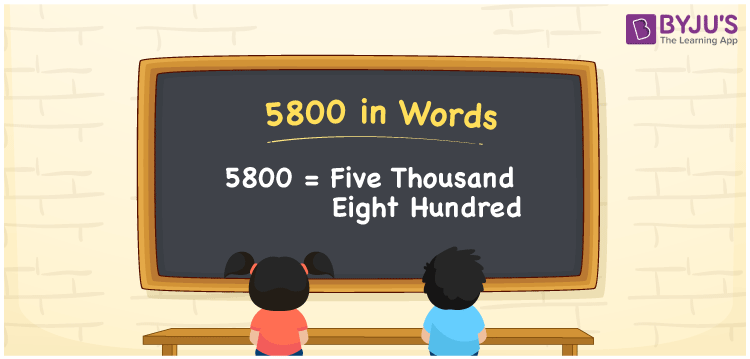# 5800 in Words

The number 5800 is represented by five thousand eight hundred. The number 5800 can be readily converted into words using the place value of a number. As a result, the number names should be written using a place value table. If you performed a transaction of Rs. 5800, you can write “I performed a transaction of Rs. Five thousand eight hundred”. The procedure for writing the number 5800 in words is explained in this article.

 5800 in Words: Five Thousand Eight Hundred. Five Thousand Eight Hundred in Numerical Form: 5800.

## 5800 in English Words## How to Write 5800 in Words?

The place value for the number 5800 is shown in the table below.

 Thousands Hundreds Tens Ones 5 8 0 0

The extended form of the number 5800 is as follows.

= 5 × Thousand + 8 × Hundred + 0 × Ten + 0 × One

= 5 × 1000 + 8 × 100

= 5000 + 800

= 5800

= Five thousand eight hundred

Hence, 5800 in words is five thousand eight hundred.

5800 in words – Five thousand eight hundred

Is 5800 an odd number? – No

Is 5800 an even number? – Yes

Is 5800 a perfect square number? – No

Is 5800 a perfect cube number? – No

Is 5800 a prime number? – No

Is 5800 a composite number? – Yes

## Frequently Asked Questions on 5800 in Words

Q1

### Write 5800 in words.

5800 in words is five thousand eight hundred.

Q2

### Simplify 5000 + 800, and express in words.

Simplifying 5000 + 800, we get 5800. Hence, 5800 in words is five thousand eight hundred.

Q3

### How to write five thousand eight hundred in numbers?

Five thousand eight hundred in numbers is 5800.